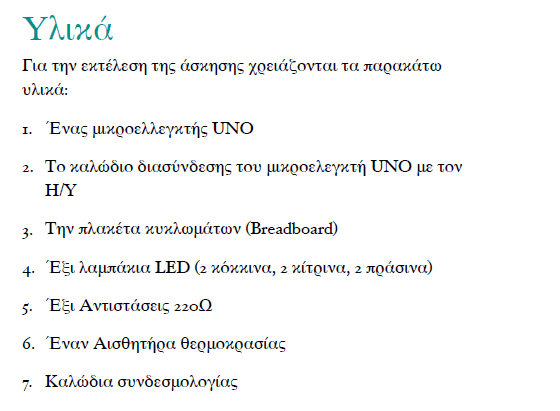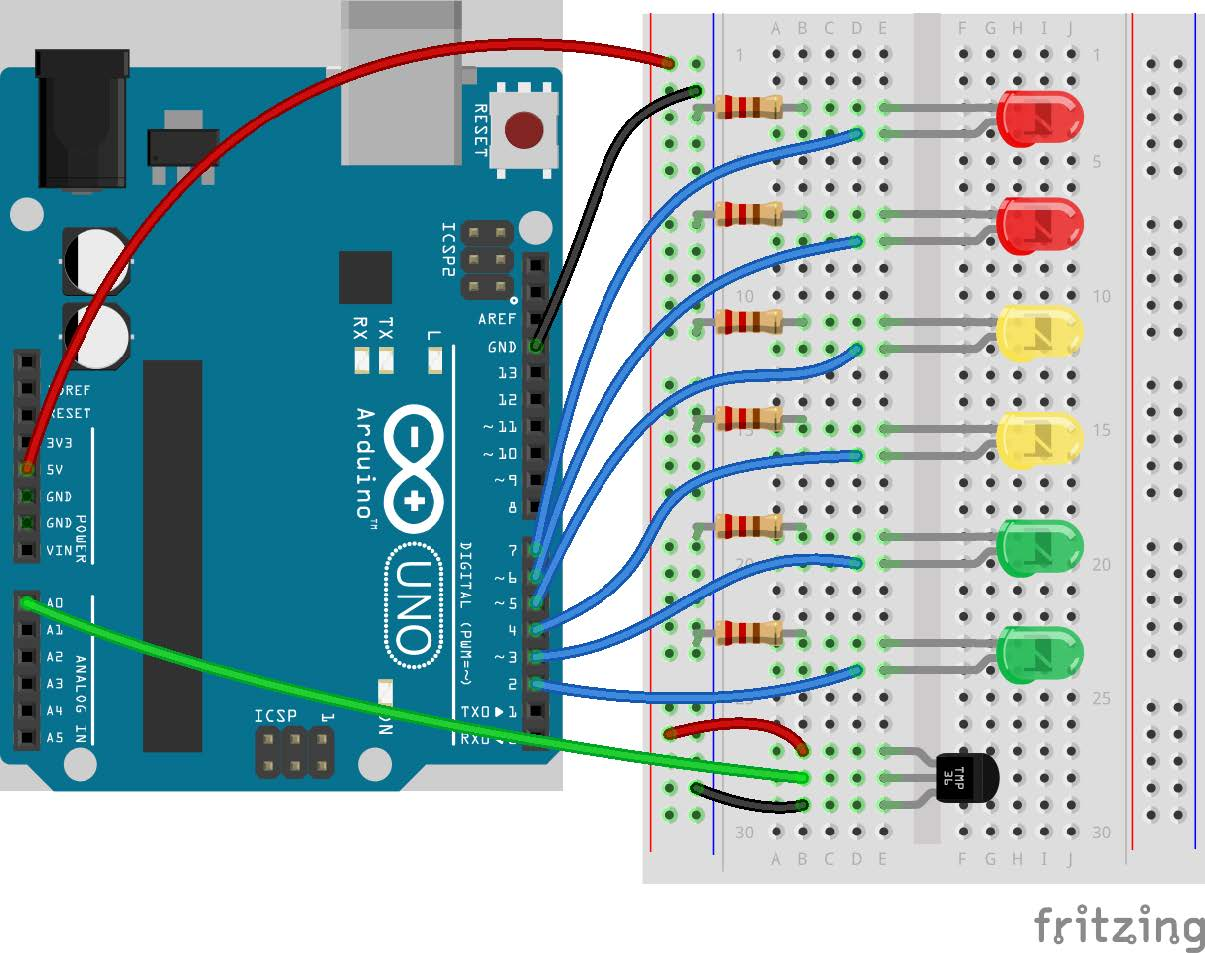tel. 24910 23755 / fax 24910 26620`/*`
`Display temperature as a color bar`
`*/`
`// constants won't change. They're used here to`
`// set pin numbers:`
`// in Pin 0 we will connect the temperature`
`// sensor. Give it a name.`
`const int tempPin = 0;`
`// in Pin 2 we will connect the low green`
`// LED. Give it a name.`
`const int lowGreenPin = 2;`
`// in Pin 3 we will connect the hi green`
`// LED. Give it a name.`
`const int hiGreenPin = 3;`
`// in Pin 4 we will connect the low yellow`
`// LED. Give it a name.`
`const int lowYellowPin = 4;`
`// in Pin 5 we will connect the hi yellow`
`// LED. Give it a name.`
`const int hiYellowPin = 5;`
`// in Pin 6 we will connect the low red`
`// LED. Give it a name.`
`const int lowRedPin = 6;`
`// in Pin 7 we will connect the hi red`
`// LED. Give it a name.`
`const int hiRedPin = 7;`
`//VARIABLES`
`float tempReading;`
`float tempVolts;`
`float tempC;`
`// the setup routine runs once when you press`
`// reset:`
`void setup() {`
`  // initialize the digital pin as an output.`
`  pinMode(lowGreenPin, OUTPUT);`
`  pinMode(hiGreenPin, OUTPUT);`
`  pinMode(lowYellowPin, OUTPUT);`
`  pinMode(hiYellowPin, OUTPUT);`
`  pinMode(lowRedPin, OUTPUT);`
`  pinMode(hiRedPin, OUTPUT);`
`  // turn off all leds at start`
`  turnOffLeds();`
`}`
`// the loop routine runs over and over again`
`// forever:`
`void loop() {`
`  // read the temperature`
`  tempReading = analogRead(tempPin);`
`  // convert reading to C`
`  tempVolts = tempReading * 5.0 / 1024.0;`
`  tempC = (tempVolts - 0.5) * 100.0;`
`  // turn off all leds`
`  turnOffLeds();`
`  // compare temperature with color scale`
`  if (tempC >= 10.00) {`
`   digitalWrite(lowGreenPin,HIGH);`
`  }`
`  if (tempC >= 15.00) {`
`   digitalWrite(hiGreenPin,HIGH);`
`  }`
`  if (tempC >= 20.00) {`
`   digitalWrite(lowYellowPin,HIGH);`
`  }`
`  if (tempC >= 25.00) {`
`   digitalWrite(hiYellowPin,HIGH);`
`  }`
`  if (tempC >= 30.00) {`
`   digitalWrite(lowRedPin,HIGH);`
`  }`
`  if (tempC >= 35.00) {`
`   digitalWrite(hiRedPin,HIGH);`
`  }`
`  delay(99);`
`}`
`void turnOffLeds() {`
`digitalWrite(lowGreenPin, LOW);`
`digitalWrite(hiGreenPin, LOW);`
`digitalWrite(lowYellowPin, LOW);`
`digitalWrite(hiYellowPin, LOW);`
`digitalWrite(lowRedPin, LOW);`
`digitalWrite(hiRedPin, LOW);`
`}`

Κατηγορίες: Arduino